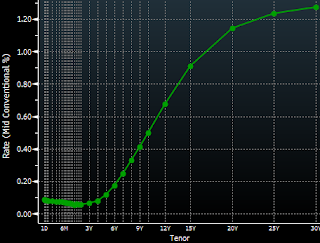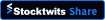## Monday, August 13, 2012

### Using LIBOR curves to discount cash flows on derivatives contracts is no longer appropriate

Some academically oriented readers may get a bit upset at this statement, but most financial derivatives pricing in practice comes down to this basic formula. The net present value (NPV) of a derivatives contract can be expressed as a sum of expected cash flows (C), weighted by the probabilities of that payout (P), discounted to valuation date using discount factors (D).

For an infinite number of possible payouts, such as an option (for example Cashflow = Max [Spot - Strike, 0]) the sum simply becomes an integral. The basic approach however remains the same.

In the past few decades the approaches to derivatives pricing focused on the cash flows and the probabilities. When it came to the discount factors, in most instances the recipe has not changed. Academic literature prescribes the building of the zero coupon LIBOR curve using BBA's LIBOR settings (at the short end), LIBOR futures, and LIBOR interest rate swaps further out. Using the so-called "bootstrapping" and some sort of interpolation technique (there is a whole science about how to interpolate for gaps in the swap curve), a zero coupon curve is built. The discount factors to be used for derivatives pricing are then derived from that that curve.

But in the past few years practitioners have been asking the question: is LIBOR really relevant for discounting derivatives cash flows? In the age of bilateral master derivatives agreements (Credit Support Annex or "CSA") when each counterparty is expected to post margin, what is the true financing cost of derivatives cash flows? The answer for most cases turns out to be quite simple. Generally the interest on collateral posted by one party to another is Fed Funds or its equivalent in another currency. The cost of funds for a derivatives contract is basically the overnight effective rate. And for most currencies there is an active market to price overnight rates expectations years into the future. It is called the Overnight Index Swap (OIS) market (see this post for more information). The chart below shows the term structure of JPY OIS for example.Yen OIS curve

Converting this OIS term structure into a zero coupon curve provides a more appropriate set of discount factors that reflect the true cost of cash flow financing. To the extent there is another collateral arrangement, the discounting should be adjusted to reflect the appropriate costs. This is already the standard for discounting interest rates swaps and is becoming common in CDS as well. Using the LIBOR curve simply because it has always been done that way is no longer appropriate, as it does not reflect the actual cost of funds. Most academic derivatives pricing literature is yet to be adjusted to reflect this fact.
JPMorgan: - Differential discounting is the practice of valuing derivative contracts using discount rates that are specific to the terms of governing collateral agreements. Using discount rates that do not reflect the terms of specific collateral agreements can misprice the full economics of derivative transactions.
...
Typically, the yield on USD cash-collateral in a CSA is based on Fed funds rather than on Libor. This means that derivative subject to collateral agreements should be values using OIS rather than Libor swap rates, which have historically been used for valuing derivative transactions. Over the past few years, the basis between Libor and OIS rates increased significantly and currently stands at 30bp in USD and 29bp in EUR for a 3-month swap. So while valuing a derivative contract using OIS rather than Libor would have had little impact in the past, over the past few years, it has had an increasing impact.

SoberLook.comBookmark this post: